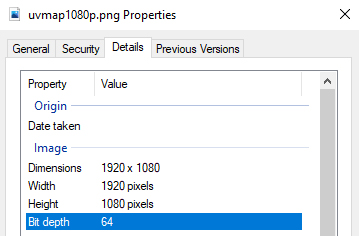/
/
vMix 虚拟集规范 1.0

# vMix 虚拟集规范 1.0

• 分类：知识库
• 作者：
• 来源：
• 发布时间：2022-05-16
• 访问量：0

【概要描述】

# vMix 虚拟集规范 1.0

【概要描述】

• 分类：知识库
• 作者：
• 来源：
• 发布时间：2022-05-16
• 访问量：0

vMix 中的虚拟集利用开放文件格式来轻松实现自定义集。

<virtualSet>
<input name="Background" x="0" y="0" zoomX="1" zoomY="1" rotateX="0" rotateY="0" rotateZ="0" cropping="0, 0,1,1" dynamic="false">Background.png</input>
<input name="Screens" x="0" y="0" zoomX="1" zoomY="1" rotateX="0 " rotateY="0" rotateZ="0" cropping="0,0,1,1" dynamic="true" uvmap="Screens.png">blank.png</input>
<input name="Talent" x ="0" y="0" zoomX="1" zoomY="1" rotateX="0" rotateY="0" rotateZ="0" 裁剪="0,0,1,1" dynamic="true" uvmap="人才。png">blank.png</input>
<zoom name="Close Up" x="0" y="0" zoom="4" />
<zoom name="Medium Shot" x="0" y= "0" zoom="2" />
<zoom name="Full" x="0" y="0" zoom="1" />
<zoom name="自定义" x="0" y="0 " zoom="1" />
</virtualSet>缩放名称="自定义" x="0" y="0" zoom="1" /> </virtualSet>缩放名称="自定义" x="0" y="0" zoom="1" /> </virtualSet>

UV 贴图图像（vMix 13 及更高版本）

UV 贴图图像经过伽马校正，每通道 16 位 PNG 文件（64 位，包括 Alpha）

### 从 Photoshop 导出 64 位深度文件

1.     创建 Photoshop 文件时，确保颜色模式设置为 16 位（16 位/通道）
2.     创建你的 UVMap
3.     要保存，请转到文件 -> 另存为（不要转到导出菜单），然后将其保存为 PNG 文件2023-08-14

2023-08-02

2023-04-21

2023-04-19

# vMix 虚拟集规范 1.0

• 分类：知识库
• 作者：
• 来源：
• 发布时间：2022-05-16
• 访问量：0

【概要描述】

# vMix 虚拟集规范 1.0

【概要描述】

• 分类：知识库
• 作者：
• 来源：
• 发布时间：2022-05-16
• 访问量：0

vMix 中的虚拟集利用开放文件格式来轻松实现自定义集。

<virtualSet>
<input name="Background" x="0" y="0" zoomX="1" zoomY="1" rotateX="0" rotateY="0" rotateZ="0" cropping="0, 0,1,1" dynamic="false">Background.png</input>
<input name="Screens" x="0" y="0" zoomX="1" zoomY="1" rotateX="0 " rotateY="0" rotateZ="0" cropping="0,0,1,1" dynamic="true" uvmap="Screens.png">blank.png</input>
<input name="Talent" x ="0" y="0" zoomX="1" zoomY="1" rotateX="0" rotateY="0" rotateZ="0" 裁剪="0,0,1,1" dynamic="true" uvmap="人才。png">blank.png</input>
<zoom name="Close Up" x="0" y="0" zoom="4" />
<zoom name="Medium Shot" x="0" y= "0" zoom="2" />
<zoom name="Full" x="0" y="0" zoom="1" />
<zoom name="自定义" x="0" y="0 " zoom="1" />
</virtualSet>缩放名称="自定义" x="0" y="0" zoom="1" /> </virtualSet>缩放名称="自定义" x="0" y="0" zoom="1" /> </virtualSet>

UV 贴图图像（vMix 13 及更高版本）

UV 贴图图像经过伽马校正，每通道 16 位 PNG 文件（64 位，包括 Alpha）

### 从 Photoshop 导出 64 位深度文件

1.     创建 Photoshop 文件时，确保颜色模式设置为 16 位（16 位/通道）
2.     创建你的 UVMap
3.     要保存，请转到文件 -> 另存为（不要转到导出菜单），然后将其保存为 PNG 文件京ICP备2021033923号-1